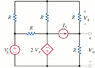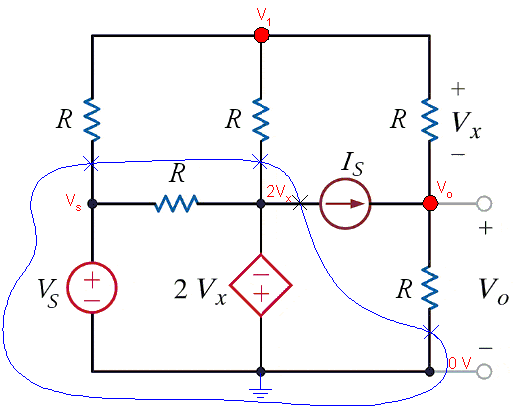# Thevenin Resistance complex circuit with dependent sources

• Engineering
Jd303
I am attempting to find Rth in the circuit attached however, I am totally lost. My work gets so messy and i seem to run around in circles. Can someone please explain to me how they would do this problem. Any help would be greatly appreciated

#### Attachments

•thev3.gif
9.9 KB · Views: 738

Homework Helper
I'd do it the way the textbooks say ... open circuit current sources and close circuit voltage sources ... redraw the resulting circuit. If I'm reading that right, one of the resistors in in parallel with a short - so you can remove it.

scootypuffsnr
i think for thevenin's with a dependent source, you first have to find vth and then you s/c where vth is and then find the current through I-s/c
after that then you get Rth = Vth/I-s/c

Hi jd303, have you learned nodal and mesh analysis yet?

With this circuit you've basically got two options.

1. Leaving all the sources intact, firstly solve to find the open circuit voltage at "Vo", and then solve once more with the output terminals shorted to find the short circuit current. The open circuit voltage is your V_thv and the ratio of V_oc/I_sc is your Z_thv.

OR

2. Set the independent sources to zero and apply an external 1 amp independent current source between the output terminals (directed toward the Vo +ive). Analyze using nodal analysis to determine the output voltage. The Thevenin resistance is numerically equal to this voltage.

Method 2, in my opinion, is the easiest way to solve this if you're trying to find R_thv alone. I got it down to just 2 nodes (apart from the ref node) and three equations.

Jd303
Hey uart,
First of all thank-you for the explanation. Secondly yes I have covered nodal and mesh analysis as well as superposition.

When Vs = 6, R=4kohms, and Is=1mA the answer is 2.9kohms

I am still yet to get this, it may be due to some small error.

I have labelled my nodes as follows and have attemped to find the open circuit voltage:
Node 1: left-hand side
Node 2: Top
Node 3: Middle
Node 4: Right - hand side
and ref node at the bottom.

Straight away i have put V1 as 6V

After nodal analysis I finish with this
3(V2) - (V3) - (V4) = 6
-3(V2) + 2(V3) + 2(V4) = 2
-V2 + 2V4 = 4

If at all possible could you please point out my error, or explain how you were left with only 2 nodes and a reference, it would be greatly appreciated! Thanks

Mentor
In nodal analysis, nodes that are directly connected by voltage sources are amalgamated into supernodes. The reference node is not immune to this procedureJd303
Really sorry guys, just can not get this one out. Can someone please show me their node equations or other method. I keep getting the wrong answer for some reason. Thanks for your patience

Mentor
Voltage sources between nodes form supernodes. Even controlled sources participate, and the reference node is not immune to this treatment.

A supernode can be identified as follows, leaving the indicated independent nodes:You should be able to write the node equations, keeping in mind that Vx is equal to V1 - Vo.

What do you get for the output voltage? How about the short-circuit current?

You can start by showing us the node equations for the first case.

#### Attachments

Jd303
Ok so i get:
Node 1: (Vs-V1)/R - (V1-2Vx)/R - (V1-Vo)/R = 0
Node 2: (V1-Vo)/R + Is - Vo/R

Which leaves me with V1 - Vo = Vs
and -V1 + 2Vo = Is*R

Have I done anything wrong?

Mentor
Ok so i get:
Node 1: (Vs-V1)/R - (V1-2Vx)/R - (V1-Vo)/R = 0
Node 2: (V1-Vo)/R + Is - Vo/R = 0
The above looks okay. Note that I completed the second equation (red bit).
Which leaves me with V1 - Vo = Vs
and -V1 + 2Vo = Is*R

Have I done anything wrong?

I think you have a sign issue with the first expression of the pair. The second looks okay.

Fix up the sign issue and solve for Vo.

Then, short the output and using a similar approach find the short-circuit current, Io.

Jd303
Will Io not just equal Is if the output is shorted?

Will Io not just equal Is if the output is shorted?

No, that would only be the case if we could assume the the current through the top resistor (the one marked with Vx) was zero. The current through that resistor is (V1-Vo)/R, which is not necessarily zero.

This is the alternative method that I mentioned previously.

The circuit simplifies down quite a lot. The resistor in parallel with the voltage source goes. Then you can do a Norton's source transformation on dependent source and combine some parallel resistors to end up with a circuit having only three resistors and two nodes.

R_thv is numerically equal to Vo in the following circuit.

#### Attachments

Jd303
Ok so I have tried attempted the theory used from gneill.

My final equations are:
V1 + Vo = Vs
-V1 + 2Vo = IsR

Therefore:
Vo = (Vs + IsR)/3
V1 = (2Vs - IsR)/3

And short circuit current equates to:
Io = Is + (V1-Vo)/R

Rth = Vo/Io

Using this I still come up with the wrong answer, can anyone see where I have gone wrong??

Once again thanks for the explanations, I feel like I am learning a lot even though i still can't get this one out

Mentor
Ok so I have tried attempted the theory used from gneill.

My final equations are:
V1 + Vo = Vs
-V1 + 2Vo = IsR

Therefore:
Vo = (Vs + IsR)/3
V1 = (2Vs - IsR)/3
The above looks good for the open-circuit case (finding Vo). You should point out at this point that the above expression for Vo is also Vth (the Thevenin voltage).
And short circuit current equates to:
Io = Is + (V1-Vo)/R
... and since Vo is shorted at this point ...

I didn't get those equations. I think the problem is the node marked "2Vx" in reply #8 should have been labeled as "-2Vx".

I went through this quickly just now and I got the same answer for R_Thv as I previously got using the "test source" method outlined in reply #12.

Mentor
I didn't get those equations. I think the problem is the node marked "2Vx" in reply #8 should have been labeled as "-2Vx".
Egads! You're right. Very careless of me, and good catch on your part.
I went through this quickly just now and I got the same answer for R_Thv as I previously got using the "test source" method outlined in reply #12.

Egads! You're right. Very careless of me, and good catch on your part.
Yeah no problems. It's always the simple mistakes that catch me out too.Jd303
uart, using replacing the centre node with -2Vx I get the following equations:

5(V1) -3(Vo) = Vs
-V1 + 2Vo + IsR

Io = Is + (V1 - Vo)/R

Rth = Vo/Io

Using the initial values set in comment #5 do you get Rth = 2.9kohms? I instead simply end up with the value of R

Jd303
Or of coarse if anyone else can see my error, as i still can not get the correct answer to this problem it would be greatly appreciated. Thanks!

Mentor
uart, using replacing the centre node with -2Vx I get the following equations:

5(V1) -3(Vo) = Vs
-V1 + 2Vo + IsR <----- not an equation

Io = Is + (V1 - Vo)/R <------ Solving for short circuit current, Vo should be zero

Rth = Vo/Io

Using the initial values set in comment #5 do you get Rth = 2.9kohms? I instead simply end up with the value of R

Once you've fixed up the above, solve the first pair for Vo in terms of Is and Vs.

When you change the circuit by shorting out the output (to find the short-circuit current), you're left with what is effectively a one independent node circuit. So you need to write a new node equation to find this new V1.

Jd303
Of coarse! Vo is not the same when the output is shorted, what an oversight! I didn't even pick that up from the hint that you tried to give me.

Thanks, I don't think i could possibly get this wrong now (But you never know)